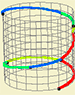## Paths on a surface### Product[see app]

If for one hour we cover one path and for the next hour we cover other path, what can we do if we want to cover both paths in one hour? The answer is simple: we have to follow on the same curves, but faster! The first path, which has been covered in hour, now could be covered in half an hour, such that we have half an hour remaining for the second path.

This is the principle for the product of two paths. Given two contiguous paths, that is where the end point of the first is the start point of the second, the path obtained from their product consists of covering both consecutively at twice the velocity. Note that the product path being continuous is a consequence of the two original paths being contiguous, and the increase on the velocity on covering the paths is needed to keep the same domain for the product path.

Matematically, we say that given two paths $$f_{1}$$ and $$f_{2}$$ from $$[0,1]$$ to $$S$$, with $$f_{2}(0)=f_{1}(1)$$, $$g=f_{1}.f_{2}$$ is a path from $$[0,1]$$ to $$S$$ such that: $g(t)=\begin{cases} \begin{array}{lcc} f_{1}(2t) & \mbox{if} & 0\leq t\leq0.5\\ f_{2}(2(t-0.5)) & \mbox{if} & 0.5<t\leq1 \end{array} & =\end{cases}\\=\begin{cases} \begin{array}{lcc} f_{1}(2t) & \mbox{if} & 0\leq t\leq0.5\\ f_{2}(2t-1) & \mbox{if} & 0.5<t\leq1 \end{array}\;\;\;\;\end{cases}$

Considering the graded rubber band interpretation, the question we have in hands is how to reduce to a single rubber band the two contiguous original rubber bands. The answer is simple: we have to stretch the rubber bands! In the apps that follow, the stretch of the rubber band is visible through the change on coloration. Assuming that we start with identical rubber bands, if a color can be seen on a bigger arc this means that the rubber band is stretched in this area.

On our description above the velocity is changed to the double of the original for each path, however this does not necessarily needs to be the case. To cover the product path in one hour, we could, for example, cover the first path in three quarters of an hour and the second in 15mins... Here, the ratio between the velocities wouldn't be the same; it wouldn't be of $$2:1$$ for both paths, but instead of $$4:3$$ for the first path and of $$4:1$$ for the second, and the product path would have been different from the one we have obtained in the description above! Nevertheless, both resulting paths are homotopic (through an homotopy of coloring). The ratio $$2:1$$ is chosen preferably for being a more natural option.

Next we study three basic properties of this product on homotopy classes.

Associativity

Neutral element

Inverse element